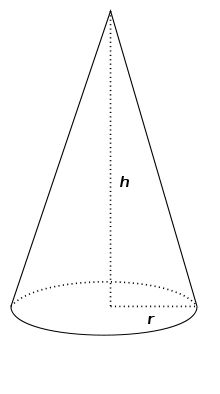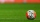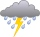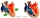# Volume of the cone

Find the volume of the cone with the base radius r and the height v.
a) r = 6 cm, v = 8 cm
b) r = 0,9 m, v = 2,3 m
c) r = 1,4 dm, v = 30 dm

Result

V1 =  301.593 cm3
V2 =  1.951 m3
V3 =  61.575 dm3

#### Solution:

$r_{1}=6 \ \text{cm} \ \\ v_{1}=8 \ \text{cm} \ \\ \ \\ V_{1}=\dfrac{ 1 }{ 3 } \cdot \ \pi \cdot \ r_{1}^2 \cdot \ v_{1}=\dfrac{ 1 }{ 3 } \cdot \ 3.1416 \cdot \ 6^2 \cdot \ 8 \doteq 301.5929 \doteq 301.593 \ \text{cm}^3$
$r_{2}=0.9 \ \text{m} \ \\ v_{2}=2.3 \ \text{m} \ \\ \ \\ V_{2}=\dfrac{ 1 }{ 3 } \cdot \ \pi \cdot \ r_{2}^2 \cdot \ v_{2}=\dfrac{ 1 }{ 3 } \cdot \ 3.1416 \cdot \ 0.9^2 \cdot \ 2.3 \doteq 1.9509 \doteq 1.951 \ \text{m}^3$
$r_{3}=1.4 \ \text{dm} \ \\ v_{3}=30 \ \text{dm} \ \\ \ \\ V_{3}=\dfrac{ 1 }{ 3 } \cdot \ \pi \cdot \ r_{3}^2 \cdot \ v_{3}=\dfrac{ 1 }{ 3 } \cdot \ 3.1416 \cdot \ 1.4^2 \cdot \ 30 \doteq 61.5752 \doteq 61.575 \ \text{dm}^3$Our examples were largely sent or created by pupils and students themselves. Therefore, we would be pleased if you could send us any errors you found, spelling mistakes, or rephasing the example. Thank you!

Leave us a comment of this math problem and its solution (i.e. if it is still somewhat unclear...):Be the first to comment!Tips to related online calculators
Tip: Our volume units converter will help you with the conversion of volume units.

## Next similar math problems:

1. Cone from cubeThe largest possible cone was turned from a 20 cm high wooden cube. Calculate its weight if you know that the density of wood was 850 kg/m3
2. Volume and surface areaFind the volume and surface of a wooden block with dimensions: a = 8 cm, b = 10 cm, c = 16 cm.
3. Triangular prismThe base of the perpendicular triangular prism is a rectangular triangle with a hypotenuse of 10 cm and one leg of 8 cm. The prism height is 75% of the perimeter of the base. Calculate the volume and surface of the prism.
4. EmbankmentThe railway embankment 300 m long has a cross section of an isosceles trapezoid with bases of 14 m and 8 m. The trapezoidal arms are 5 m long. Calculate how much m3 of soil is in the embankment?
5. What is bigger?Which ball has a larger volume: a football with a circumference of 66 cm or a volleyball with a diameter of 20 cm?
6. Oak trunkCalculate in tonnes the approximate weight of a cylindrical oak trunk with a diameter of 66 cm and a length of 4 m, knowing that the density of the wood was 800 kg/m³.
7. CannonballsOf the three cannonballs with a diameter of 16 cm, which landed in the castle courtyard during the battle, the castle blacksmith cast balls with a diameter of 10 cm, which fit into the cannons placed on the walls. How many cannonballs did the blacksmith c
8. The copper wireThe copper wire bundle with a diameter of 2.8mm has a weight of 5kg. How many meters of wire is bundled if 1m3 of copper weighs 8930kg?
9. Two rectangular boxesTwo rectangular boxes with dimensions of 5 cm, 8 cm, 10 cm, and 5 cm, 12 cm, 1 dm are to be replaced by a single cube box of the same cubic volume. Calculate its surface.
10. Diameter = heightThe surface of the cylinder, the height of which is equal to the diameter of the base, is 4239 cm square. Calculate the cylinder volume.
11. Triangular prism - regularThe regular triangular prism is 7 cm high. Its base is an equilateral triangle whose height is 3 cm. Calculate the surface and volume of this prism.
12. The EarthThe Earth's surface is 510,000,000 km2. Calculates the radius, equator length, and volume of the Earth, assuming the Earth has the shape of a sphere.
13. Hemisphere cutCalculate the volume of the spherical layer that remains from the hemisphere after the 3 cm section is cut. The height of the hemisphere is 10 cm.
14. RainGarden shape of a rectangle measuring 15 m and 20 m rained water up to 3 mm. How many liters of water rained on the garden?
15. Permille of alcoholI have 2 per mille of alcohol in my blood. How many milliliters is it when I have 5 liters of blood?
16. KostkaKostka je vepsána do koule o poloměru r = 6 cm. Kolik procent tvoří objem kostky z objemu koule?
17. Block or cuboidThe wall diagonals of the block have sizes of √29cm, √34cm, √13cm. Calculate the surface and volume of the block.